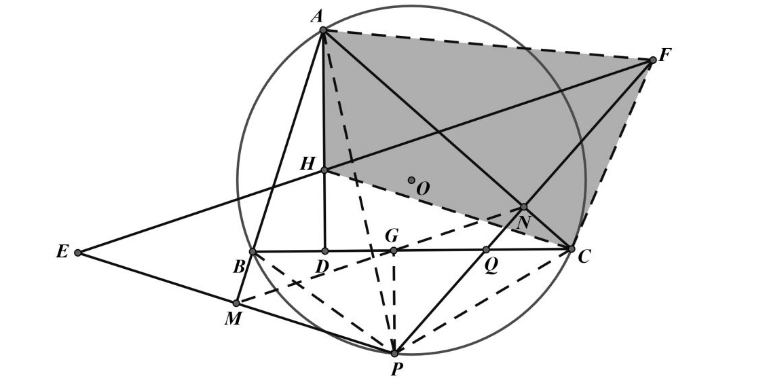【答案】

$\angle M B P=\angle A C P, \angle M G B=\angle M P B=\angle N P C=\angle N G C$,
$\therefore M 、 G 、 N$ 三点共线。 $M N / / E F, A 、 D 、 Q 、 N$ 四点共圆,
$\angle D A C=\angle N Q C=\angle N P C+\angle P C Q=\angle N F C+\angle P N G=\angle N F C+\angle H F P=\angle H F C$
$\therefore H 、 A 、 F 、 C$ 四点共圆, $\angle D H C=\angle A F C=\angle A P C=\angle A B C$
$\therefore \angle A B C+\angle B C H=\angle D H C+\angle B C H=90^{\circ}$
$\therefore C H \perp A B, H$ 是 $\triangle A B C$ 的垂心。